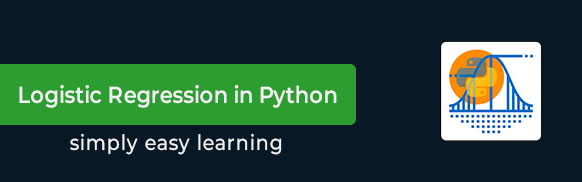# Logistic Regression in Python Tutorial

Logistic Regression is a statistical method of classification of objects. In this tutorial, we will focus on solving binary classification problem using logistic regression technique. This tutorial also presents a case study that will let you learn how to code and apply Logistic Regression in Python.

# Audience

This tutorial has been prepared for students as well as professionals to gain a knowledge on performing Logistic Regression in Python.

# Prerequisites

This tutorial is written with an assumption that the learner is familiar with Python and its libraries, such as Pandas, Numpy, and Matplotlib. If you are new to Python or these libraries, we suggest you pick a tutorial based on them before you start your journey with Logistic Regression.